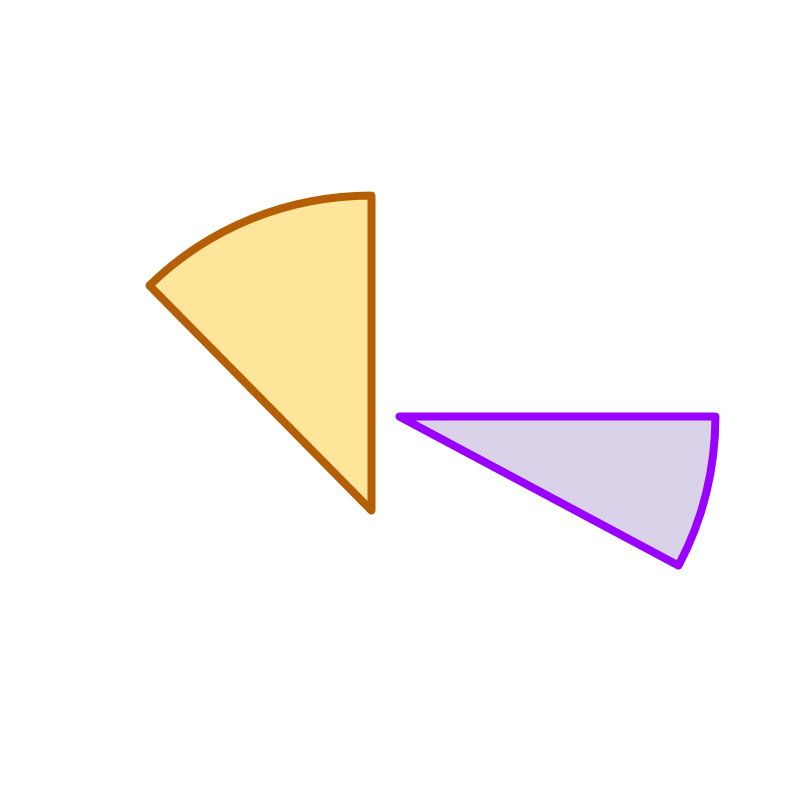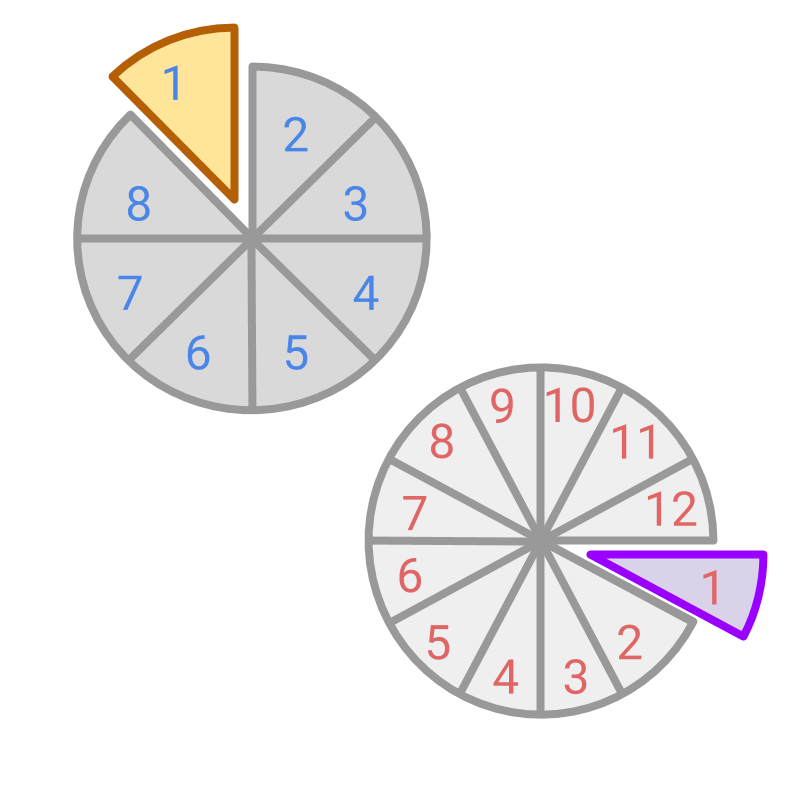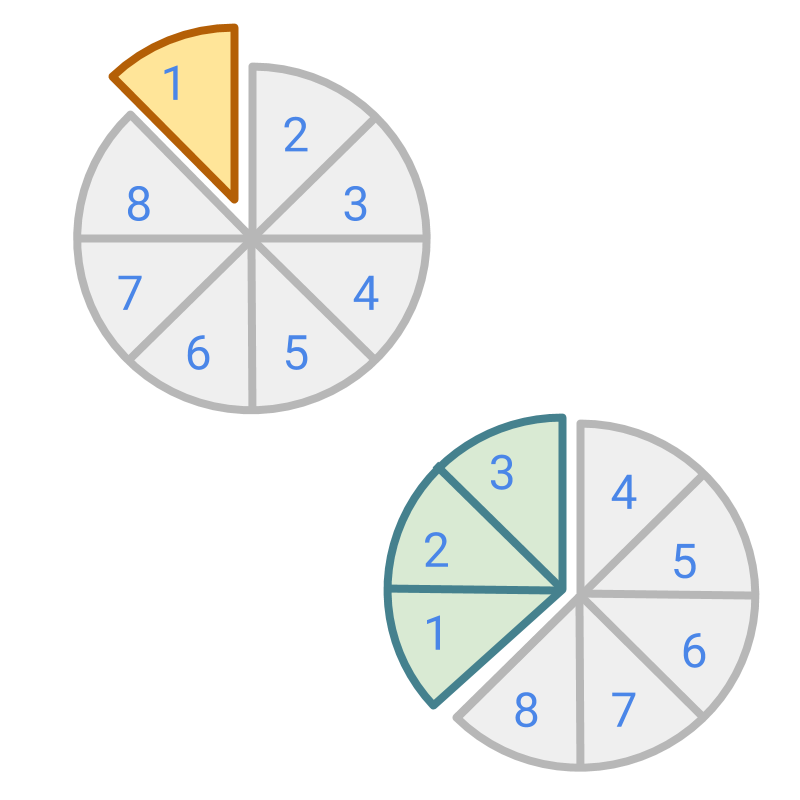maths > fractions

Like and Unlike fractions

what you'll learn...

overview

This page introduces like and unlike fractions based on the denominators of the fractions.

•  Like fractions are fractions in the same place value and so denominators are equal.

•  Unlike fractions are fractions in different place values and so denominators are not equal.

all are not alikeConsider the two fractions represented in the figure. Both are of the same size and so they are equal.The fractions represented in the figure are of different amounts.

Though the two fractions are shown as one piece each, they represent two different fractions.The figure shows the two fractions as part of their whole. In the first fraction, the whole is divided into $8$$8$ pieces and the fraction represents $\frac{1}{8}$$\frac{1}{8}$ place value. In the second fraction, the whole is divided into $12$$12$ pieces and the fraction represents $\frac{1}{12}$$\frac{1}{12}$ place value.

The sizes of single pieces are not equal. That is reflected in the denominator, as the denominator represents the number of parts the whole is divided into or the place value.

The figure shows the two fractions with different place values. First fraction has the place value of $\frac{1}{8}$$\frac{1}{8}$ and the second has the place value of $\frac{1}{12}$$\frac{1}{12}$. That is reflected in the denominators of the fractions, as the denominator represents the place value.

like and unlike

Like fractions are fractions in the same place value and so denominators are equal.

Unlike fractions are fractions in different place values and so denominators are not equal.

Like Fractions: The fractions are given in the same place value and so the denominators are equal.
eg: $\frac{2}{4}$$\frac{2}{4}$ and $\frac{3}{4}$$\frac{3}{4}$.

Unlike Fractions: The whole is divided into parts of different sizes. The fractions given in different place values and so the denominators are not equal.
eg: $\frac{2}{3}$$\frac{2}{3}$ and $\frac{3}{5}$$\frac{3}{5}$.

The word "like" means: having same characteristics or qualities.

The word "unlike" means having different characteristics or qualities.

What are the fractions $\frac{2}{4}$$\frac{2}{4}$ and $\frac{2}{5}$$\frac{2}{5}$?
→  eg: $\frac{1}{8}$$\frac{1}{8}$ and $\frac{3}{8}$$\frac{3}{8}$»  Unlike Fractions
→  eg: $\frac{1}{8}$$\frac{1}{8}$ and $\frac{1}{12}$$\frac{1}{12}$